This manual is for an old version of Hazelcast Jet, use the latest stable version.

Let us now introduce the basic concept of DAG-based distributed computing. We'll use a simple example that may already be familiar: there is a dataset consisting of lines of text in plain English and we want to find the number of occurrences of each word in it. A single-threaded computation that does it can be expressed in just a few lines of Java:

``````List<String> lines = ... // a pre-existing list
Map<String, Long> counts = new HashMap<>();
for (String line : lines) {
for (String word : line.split("\\W+")) {
counts.merge(word.toLowerCase(), 1L, (o, n) -> o + n);
}
}
``````

We'll move in small increments from this towards a formulation that is ready to be executed simultaneously on all machines in a cluster, utilizing all the CPU cores on each of them.

The first step will be modeling the computation as a DAG. We'll start from a single-threaded model and gradually expand it into a parallelized, distributed one, discussing at each step the concerns that arise and how to meet them. Then, in the second part, we'll show you the code to implement and run it on a Hazelcast Jet cluster.

Note that here we are describing a batch job: the input is finite and present in full before the job starts. Later on we'll present a streaming job that keeps processing an infinite stream forever, transforming it into another infinite stream.

## Modeling Word Count in terms of a DAG

The word count computation can be roughly divided into three steps:

1. Read a line from the map ("source" step).
2. Split the line into words ("tokenize" step).
3. Update the running totals for each word ("accumulate" step).

We can represent these steps as a DAG: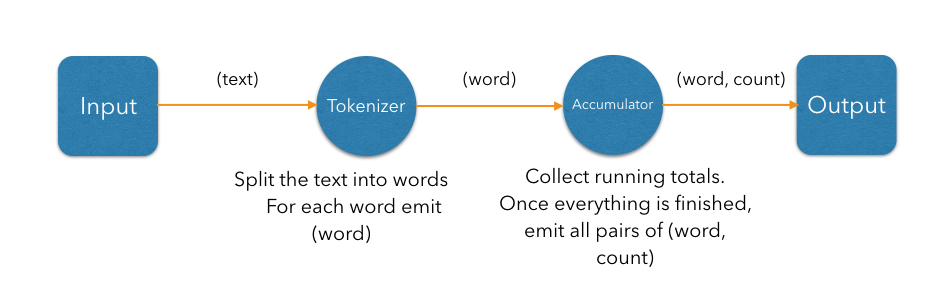In the simplest case, the computation inside each vertex can be executed in turn in a single-threaded environment; however, just by modeling the computation as a DAG, we've split the work into isolated steps with clear data interfaces between them. This means each vertex can have its own thread and they can communicate over concurrent queues: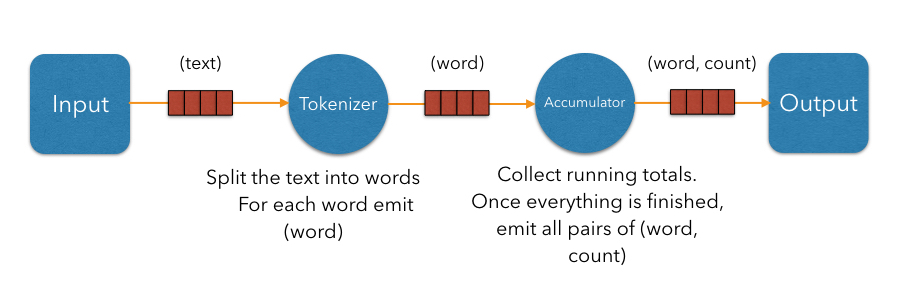This achieves a pipelined architecture: while the tokenizer is busy with the regex work, the accumulator is updating the map using the data the tokenizer is done with; and the source and sink stages are pumping the data from/to the environment. Our design is now able to engage more than one CPU core and will complete that much sooner; however, we're still limited by the number of vertices. We'll be able utilize two or three cores regardless of how many are available. To move forward we must try to parallelize the work of each individual vertex.

Given that our input is an in-memory list of lines, the bottleneck occurs in the processing stages (tokenizing and accumulating). Let's first attack the tokenizing stage: it is a so-called "embarrassingly parallelizable" task because the processing of each line is completely self-contained. At this point we have to make a clear distinction between the notions of vertex and processor: there can be several processors doing the work of a single vertex. Let's add another tokenizing processor: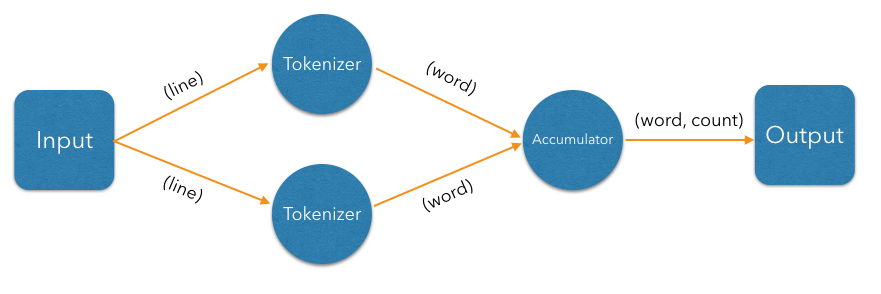The input processor can now use all the available tokenizers as a pool and submit to any one whose queue has some room.

The next step is parallelizing the accumulator vertex, but this is trickier: accumulators count word occurrences so using them as a pool will result in each processor observing almost all distinct words (entries taking space in its hashtable), but the counts will be partial and will need combining. The common strategy to reduce memory usage is to ensure that all occurrences of the same word go to the same processor. This is called "data partitioning" and in Jet we'll use a partitioned edge between the tokenizer and the accumulator: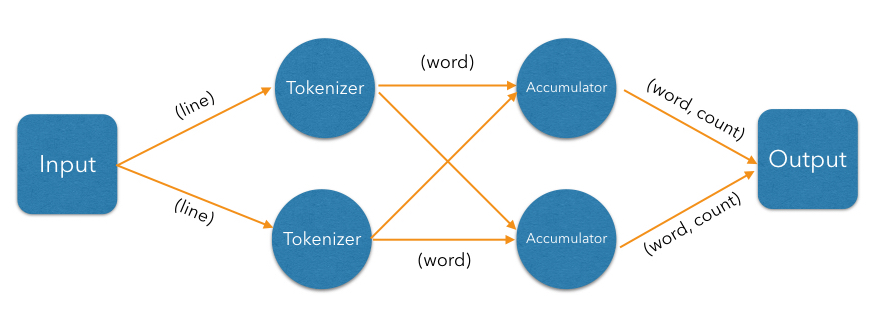As a word is emitted from the tokenizer, it goes through a "switchboard" stage where it's routed to the correct downstream processor. To determine where a word should be routed, we can calculate its hashcode and use the lowest bit to address either accumulator 0 or accumulator 1.

At this point we have a blueprint for a fully functional parallelized computation job which can max out all the CPU cores given enough instances of tokenizing and accumulating processors. The next challenge is making this work across machines.

For starters, our input can no longer be a simple in-memory list because that would mean each machine processes the same data. To exploit a cluster as a unified computation device, each cluster member must observe only a slice of the dataset. Given that a Jet instance is also a fully functional Hazelcast IMDG instance and a Jet cluster is also a Hazelcast IMDG cluster, the natural choice is to pre-load our data into an `IMap`, which will be automatically partitioned and distributed between the members. Now each Jet member can just read the slice of data that was stored locally on it.

When run in a cluster, Jet will instantiate a replica of the whole DAG on each member. On a two-member cluster there will be two source processors, four tokenizers, and so on. The trickiest part is the partitioned edge between tokenizer and accumulator: each accumulator is supposed to receive its own subset of words. That means that, for example, a word emitted from tokenizer 0 will have to travel across the network to reach accumulator 3, if that's the one that happens to own it. On average we can expect every other word to need network transport, causing both serious network traffic and serialization/deserialization CPU load.

There is a simple trick we can employ to avoid most of this traffic, closely related to what we pointed above as a source of problems when parallelizing locally: members of the cluster can be used as a pool, each doing its own partial word counts, and then a downstream vertex will combine those results. As noted above, this takes more memory due to more hashtable entries on each member, but it saves network traffic (an issue we didn't have within a member). Given that memory costs scale with the number of distinct keys, and given our specific use case with words of a natural language, the memory cost is more-or-less constant regardless of how much book material we process. On the other hand, network traffic scales with the total data size so the more material we process, the more we save on network traffic.

Jet distinguishes between local and distributed edges, so we'll use a local partitioned edge for `tokenize`->`accumulate` and a distributed partitioned edge for `accumulate`->`combine`. With this move we've finalized our DAG design, which can be illustrated by the following diagram: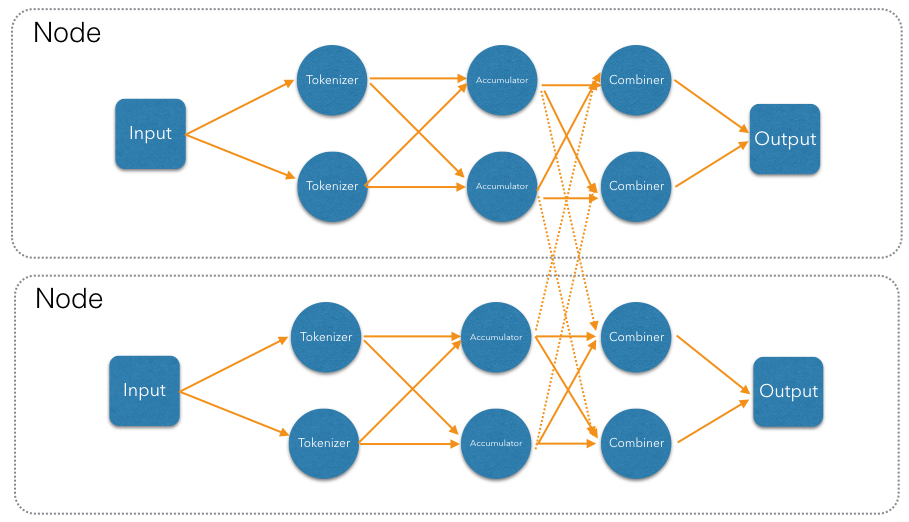## Implementing and Running the DAG

Now that we've come up with a good DAG design, it's time to implement it using the Jet DAG API. We'll present this in several steps:

1. Start a Jet cluster.
2. Populate an `IMap` with sample data.
3. Build the Jet DAG.
4. Submit it for execution.

To start a new Jet cluster, we must start some Jet instances. Typically these would be started on separate machines, but for the purposes of this tutorial we'll be using the same JVM for both instances. We can start them as shown below:

``````public class WordCount {
public static void main(String[] args) {
JetInstance jet = Jet.newJetInstance();
Jet.newJetInstance();
}
}
``````

These two instances should automatically discover each other using IP multicast and form a cluster. You should see a log output similar to the following:

``````Members  {
Member [10.0.1.3]:5701 - f1e30062-e87e-4e97-83bc-6b4756ef6ea3
Member [10.0.1.3]:5702 - d7b66a8c-5bc1-4476-a528-795a8a2d9d97 this
}
``````

This means the members successfully formed a cluster. Don't forget to shut down the members afterwards, by adding the following as the last line of your application:

``````Jet.shutdownAll();
``````

This must be executed unconditionally, even in the case of an exception; otherwise your Java process will stay alive because Jet has started its internal threads:

``````public class WordCount {
public static void main(String[] args) {
try {
JetInstance jet = Jet.newJetInstance();
Jet.newJetInstance();

} finally {
Jet.shutdownAll();
}
}
}
``````

As explained earlier, we'll use an `IMap` as our data source. Let's give it some sample data:

``````IMap<Integer, String> map = jet.getMap("lines");
map.put(0, "It was the best of times,");
map.put(1, "it was the worst of times,");
map.put(2, "it was the age of wisdom,");
map.put(3, "it was the age of foolishness,");
map.put(4, "it was the epoch of belief,");
map.put(5, "it was the epoch of incredulity,");
map.put(6, "it was the season of Light,");
map.put(7, "it was the season of Darkness");
map.put(8, "it was the spring of hope,");
map.put(9, "it was the winter of despair,");
map.put(10, "we had everything before us,");
map.put(11, "we had nothing before us,");
map.put(12, "we were all going direct to Heaven,");
map.put(13, "we were all going direct the other way --");
map.put(14, "in short, the period was so far like the present period, that some of "
+ "its noisiest authorities insisted on its being received, for good or for "
+ "evil, in the superlative degree of comparison only.");
``````

Now we move on to the code that builds and runs the DAG. We start by instantiating the DAG class and adding the source vertex:

``````DAG dag = new DAG();
``````

It will read the lines from the `IMap` and emit items of type `Map.Entry<Integer, String>` to the next vertex. The key of the entry is the line number, and the value is the line itself. The built-in map-reading processor will do just what we want: on each member it will read only the data local to that member.

The next vertex is the tokenizer, which does a simple "flat-mapping" operation (transforms one input item into zero or more output items). The low-level support for such a processor is a part of Jet's library, we just need to provide the mapping function:

``````// (lineNum, line) -> words
Pattern delimiter = Pattern.compile("\\W+");
Vertex tokenize = dag.newVertex("tokenize",
Processors.flatMap((Entry<Integer, String> e) ->
Traversers.traverseArray(delimiter.split(e.getValue().toLowerCase()))
.filter(word -> !word.isEmpty()))
);
``````

This creates a processor that applies the given function to each incoming item, obtaining zero or more output items, and emits them. Specifically, our processor accepts items of type `Entry<Integer, String>`, splits the entry value into lowercase words, and emits all non-empty words. The function must return a `Traverser`, which is a functional interface used to traverse a sequence of non-null items. Its purpose is equivalent to the standard Java `Iterator`, but avoids the cumbersome two-method API. Since a lot of support for cooperative multithreading in Hazelcast Jet deals with sequence traversal, this abstraction simplifies many of its aspects.

The next vertex will do the actual word count. We can use the built-in `accumulateByKey` processor for this:

``````// word -> (word, count)
Vertex accumulate = dag.newVertex("accumulate",
Processors.accumulateByKey(
DistributedFunctions.wholeItem(),
AggregateOperations.counting())
);
``````

This processor maintains a hashtable that maps each distinct key to its accumulated value. We specify `wholeItem()` as the key extractor function: our input item is just the word, which is also the grouping key. The second argument is the kind of aggregate operation we want to perform — counting. We're relying on Jet's out-of-the-box definitions here, but it's easy to define your own aggregate operations and key extractors. The processor emits nothing until it has received all the input, and at that point it emits the hashtable as a stream of `Entry<String, Long>`.

Next is the combining step which computes the grand totals from individual members' contributions. This is the code:

``````// (word, count) -> (word, count)
Vertex combine = dag.newVertex("combine",
Processors.combineByKey(AggregateOperations.counting())
);
``````

`combineByKey` is designed to be used downstream of `accumulateByKey`, which is why it doesn't need an explicit key extractor. The aggregate operation must be the same as on `accumulateByKey`.

The final vertex is the sink — we want to store the output in another `IMap`:

``````Vertex sink = dag.newVertex("sink", Processors.writeMap("counts"));
``````

Now that we have all the vertices, we must connect them into a graph and specify the edge type as discussed in the previous section. Here's all the code at once:

``````dag.edge(between(source, tokenize))
.edge(between(tokenize, accumulate)
.partitioned(DistributedFunctions.wholeItem(), Partitioner.HASH_CODE))
.edge(between(accumulate, combine)
.distributed()
.partitioned(DistributedFunctions.entryKey()))
.edge(between(combiner, sink));
``````

Let's take a closer look at some of the edges. First, source to tokenizer:

``````   .edge(between(tokenize, accumulate)
.partitioned(DistributedFunctions.wholeItem(), Partitioner.HASH_CODE))
``````

We chose a local partitioned edge. For each word, there will be a processor responsible for it on each member so that no items must travel across the network. In the `partitioned()` call we specify two things: the function that extracts the partitioning key (`wholeItem()` — same as the grouping key extractor), and the policy object that decides how to compute the partition ID from the key. Here we use the built-in `HASH_CODE`, which will derive the ID from `Object.hashCode()`. As long as the the definitions of `equals()/hashCode()` on the key object match our expected notion of key equality, this policy is always safe to use on a local edge.

Next, the edge from the accumulator to the combiner:

``````.edge(between(accumulate, combine)
.distributed()
.partitioned(DistributedFunctions.entryKey()))
``````

It is distributed partitioned: for each word there is a single `combiner` processor in the whole cluster responsible for it and items will be sent over the network if needed. The partitioning key is again the word, but here it is the key part of the `Map.Entry<String, Long>`. For demonstration purposes we are using the default partitioning policy here (Hazelcast's own partitioning scheme). It is the slower-but-safe choice on a distributed edge. Detailed inspection shows that hashcode-based partitioning would be safe as well because all of `String`, `Long`, and `Map.Entry` have the hash function specified in their Javadoc.

To run the DAG and print out the results, we simply do the following:

``````jet.newJob(dag).execute().get();
System.out.println(jet.getMap("counts").entrySet());
``````

The final output should look like the following:

``````[heaven=1, times=2, of=12, its=2, far=1, light=1, noisiest=1,
the=14, other=1, incredulity=1, worst=1, hope=1, on=1, good=1, going=2,
like=1, we=4, was=11, best=1, nothing=1, degree=1, epoch=2, all=2,
that=1, us=2, winter=1, it=10, present=1, to=1, short=1, period=2,